# please help! aw a S Using an aggregate demand and supply graph, show and describe the...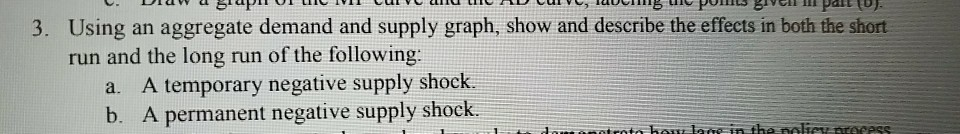aw a S Using an aggregate demand and supply graph, show and describe the effects in both the short run and the long run of the following: 3. a. A temporary negative supply shock. b. A permanent negative supply shock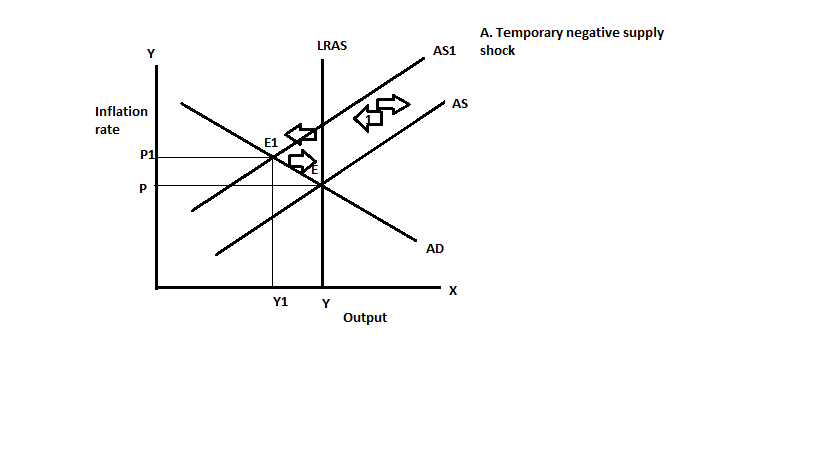In the short run a temporary negative shock will shif the aggregate supply curve to the upward, causes an increase in the inflation and decrease in the output. But in the long run the economy will be back to its original position, with the output is less than the potential output the expected inflation declines so this now shifts the aggregate supply curve to the downward. So now the economy in is back to its original position. The shifts in the aggregate supply curve and the equilibrium is indicated by the arrows.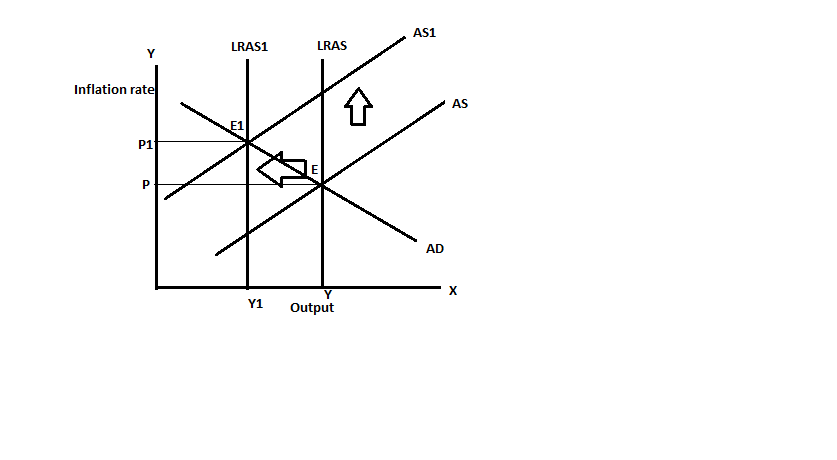The effects of the permanent negative shock is graphed above, a negative supply shock would cause the short run aggregate supply curve shift to the upward and the long run aggregate supply curve shifts to the left. In the long run there would be permanent rise in the inflation and the decline in the output.

Similar Homework Help Questions
• ### 5. (io points) Describe the effects of the following phenomena in both the short run and...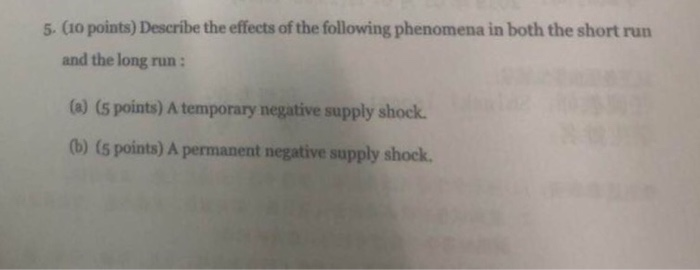5. (io points) Describe the effects of the following phenomena in both the short run and the long run: (a) (s points) A temporary negative supply shock. 0b) (s points) A permanent negative supply shock.

• ### • draw an aggregate demand and aggregate supply diagram to illustrate your answer • show the...

• draw an aggregate demand and aggregate supply diagram to illustrate your answer • show the change in aggregate demand and/or aggregate supply • describe the change(s) you have shown • explain why the adjustments you have described occur. 1. Suppose that there is an expansion of private consumption due to increased optimism about future growth prospects for the economy. (i) Illustrate and explain the effect of this shock in the short-run. (ii) What is the long-run effect likely to...

• ### 1. (s points) "The Fed can perfectly control the supply of reserves in the market Is...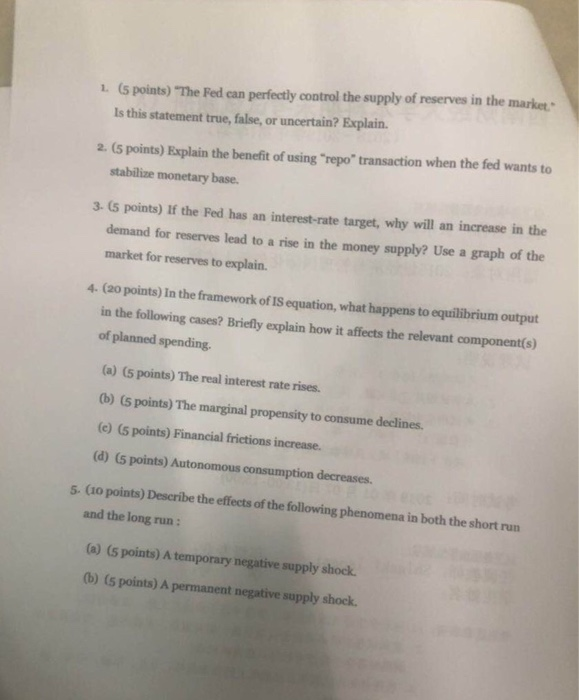1. (s points) "The Fed can perfectly control the supply of reserves in the market Is this statement true, false, or uncertain? Explain. 2. (5 points) Explain the benefit of using "repo" transaction when the fed wants to stabilize monetary base. 3. (5 points) If the Fed has an interest-rate target, why will an increase in the demand for reserves lead to a rise in the money supply? Use a graph of the market for reserves to explain. 4 (2o...

• ### Unit 3: Aggregate Demand, Aggregate Supply, and Fiscal Policy AD, AS, and LRAS Short Run vs....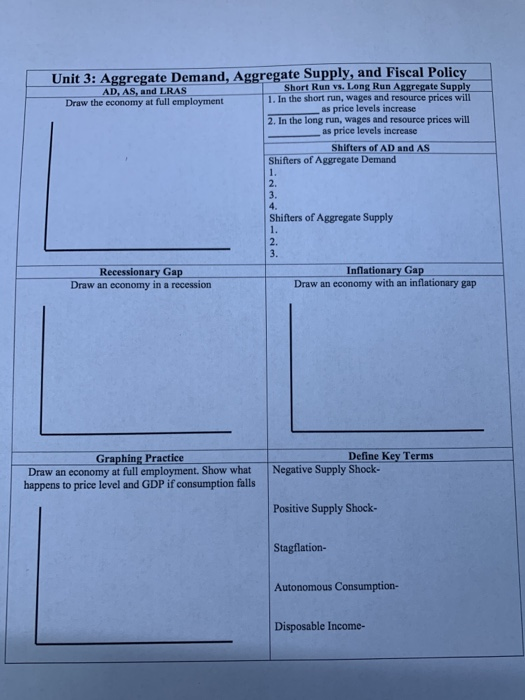Unit 3: Aggregate Demand, Aggregate Supply, and Fiscal Policy AD, AS, and LRAS Short Run vs. Long Run Aggregate Supply Draw the economy at full employment 1. In the short run, wages and resource prices will as price levels increase 2. In the long run, wages and resource prices will as price levels increase Shifters of AD and AS Shifters of Aggregate Demand Shifters of Aggregate Supply imi Recessionary Gap Draw an economy in a recession Inflationary Gap Draw an...

• ### Beginning with long-run equilibrium, use the aggregate demand and aggregate supply model to illustrate what happens...

Beginning with long-run equilibrium, use the aggregate demand and aggregate supply model to illustrate what happens in the short run when the economy suffers a negative supply shock. (10 points)

• ### A supply shock causes a shift in: a. long-run aggregate supply. b. aggregate demand. c. short-run and long-run aggregat...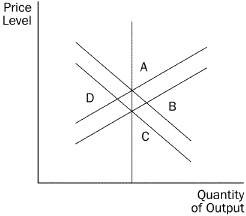A supply shock causes a shift in: a. long-run aggregate supply. b. aggregate demand. c. short-run and long-run aggregate supply. d. short-run aggregate supply. e. aggregate demand and short-run aggregate supply. Consider the exhibit below for the following questions. Figure 20-1 Refer to Figure 20-1. The economy would be moving to long-run equilibrium if it started at a. A and moved to B. b. C and moved to B. c. D and moved to C. d. None of the above...

• ### Using the IS-LM and Aggregate Supply-Aggregate Demand (AS-AD) models of Chapter 12 with a flat short-run...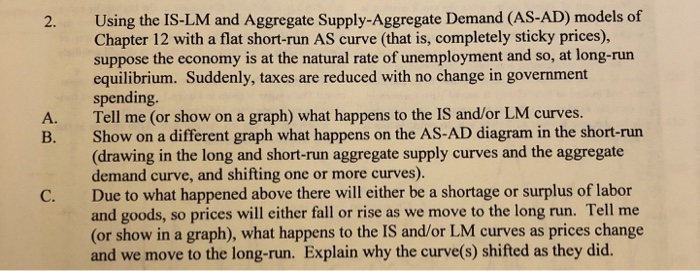Using the IS-LM and Aggregate Supply-Aggregate Demand (AS-AD) models of Chapter 12 with a flat short-run AS curve (that is, completely sticky prices), suppose the economy is at the natural rate of unemployment and so, at long-run equilibrium. Suddenly, taxes are reduced with no change in government spending. Tell me (or show on a graph) what happens to the IS and/or LM curves. Show on a different graph what happens on the AS-AD diagram in the short-run (drawing in the...

• ### *This is from the most recent quarter of 2019* Draw and carefully describe a graph that utilizes the Aggregate Demand/Ag...

*This is from the most recent quarter of 2019* Draw and carefully describe a graph that utilizes the Aggregate Demand/Aggregate Supply model that would illustrate the current state of the aggregate economy in the United States. Your graph needs to be clearly labeled and explained in some detail. Make sure that your graph includes an aggregate demand (AD) curve, a short run aggregate supply (SRAS) curve, and a long run aggregate supply curve (LRAS, Potential GDP) curve. You should clearly...

• ### Aggregate supply and aggregate demand in Lithuania were in their long run equilibrium. Then consumers decided...

Aggregate supply and aggregate demand in Lithuania were in their long run equilibrium. Then consumers decided to spend less and save more. In a well-labeled graph, show how aggregate demand, aggregate supply, and the equilibrium change in both the short and long run (6 points). Explain what happened to the economy, especially the price level and output, in the short and long run (2 points). Show (in a pair of graphs) what the central bank could do to offset the...

• ### Please help with both questions The short-run aggregate supply curve is horizontal when Othere are unemployed...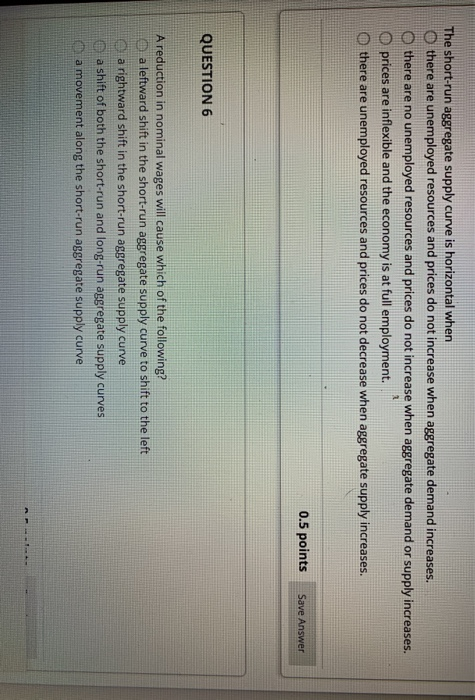Please help with both questions The short-run aggregate supply curve is horizontal when Othere are unemployed resources and prices do not increase when aggregate demand increases. there are no unemployed resources and prices do not increase when aggregate demand or supply increases. prices are inflexible and the economy is at full employment. Othere are unemployed resources and prices do not decrease when aggregate supply increases. 0.5 points Save Answer QUESTION 6 A reduction in nominal wages will cause which of...

Free Homework App